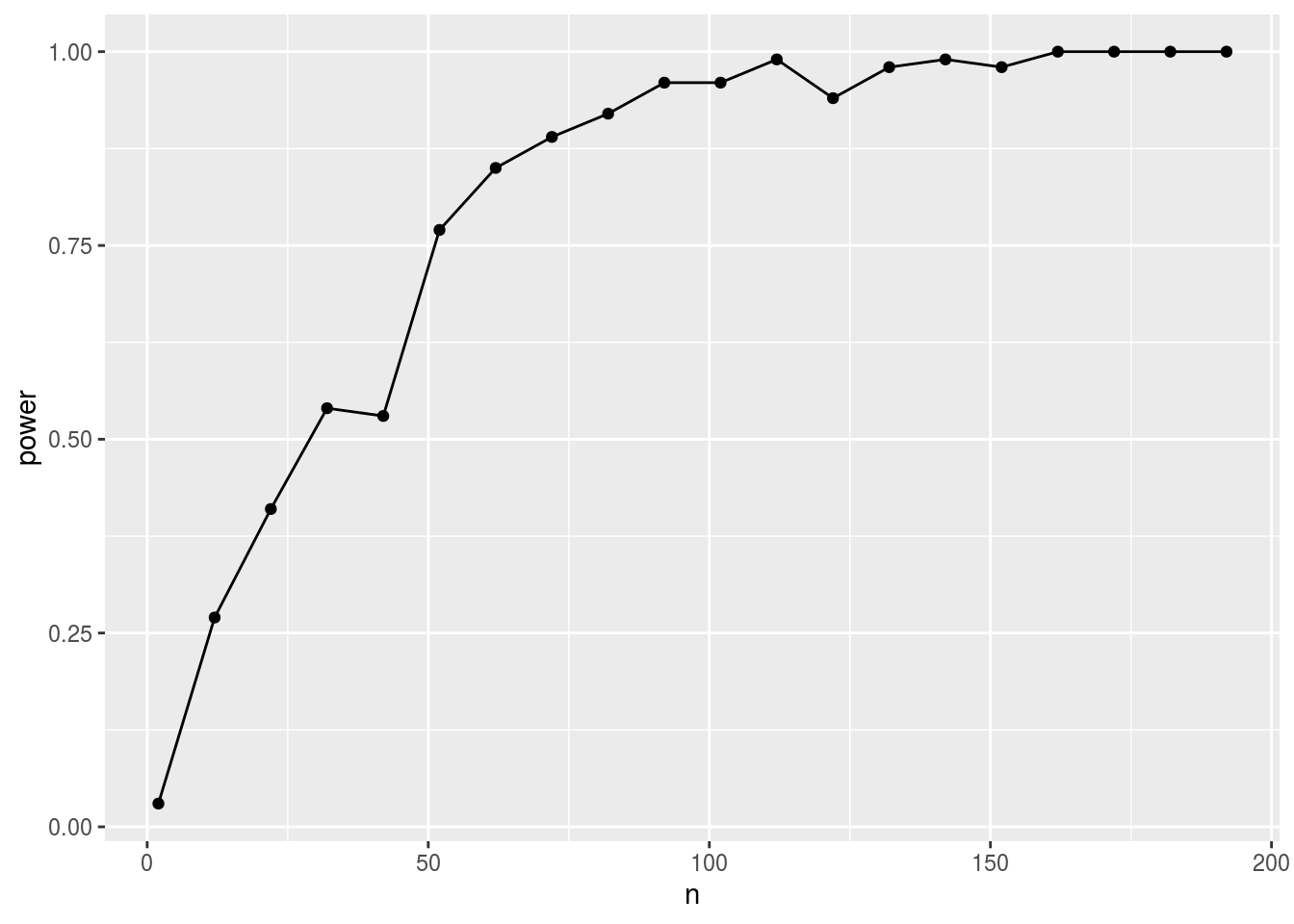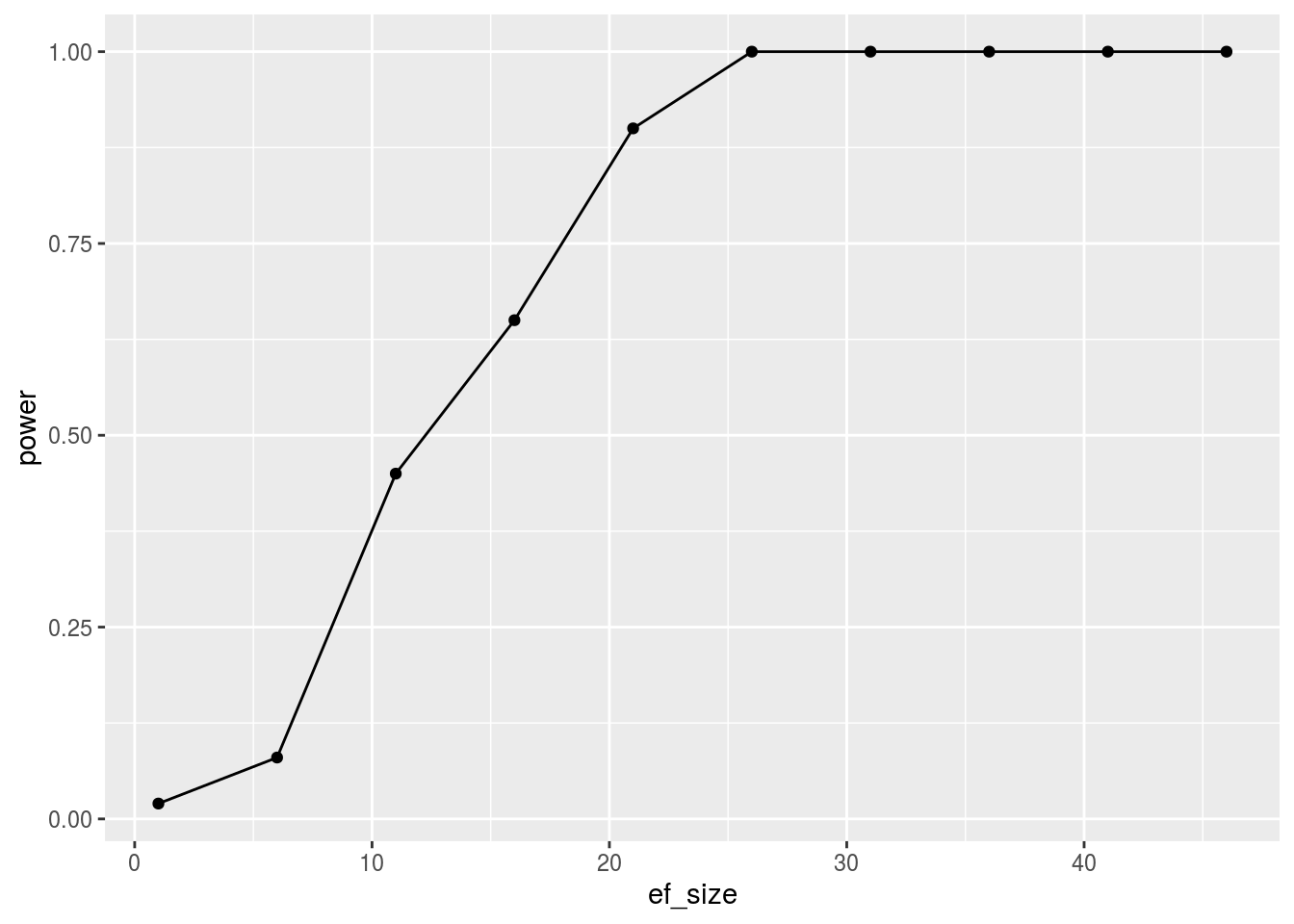# What is statistical power?

Last time, we talked about how to conduct hypothesis testing with the z-distribution and we talked about how to calculate the statistical significance (obtained probability) of any given effect. Today, we are going to talk about statistical power. Statistical power is the probability of rejecting the null hypothesis, when the null hypothesis is false (or when there is an effect). Another way of saying this: imagine if you sampled the whole population and conducted your experiment, what is the size of the effect? We’ll use simulations to explore the concept of statistical power. We’ll run multiple experiments with samples drawn from known distributions and then we’ll calculate how many of those experiments are significant. This is the core idea of statistical power.

Let’s simulate a very simple experiment like the one we did with hypothesis testing. Here, we will draw two samples. This time however, we will add an effect size parameter: it is the “true” difference between the null group (who got the placebo pill) and the experimental group (who got the memory-improving pill). An effect size here simply means the size of the effect of this pill. The true difference then should be equal to the effect size (we are no longer talking about a null difference).

The question now is this: if I think my pill has this effect size, and if I am assuming that scores are drawn from normal distributions with a sample size of 20, and if my alpha (probability of rejecting the null when it is false: Type I Error) is set to 5%: what is the probability that I’ll reject the null hypothesis? This is a fair question and can be easily answered as we’ll see below.

The only difference here is that instead of using the mean differences method, I am not using a t.test that is very similar to the mean differences method we discussed last class. We’ll take the p-value of this t-test and only record if it is significant.

# Using simulations to understand statistical power

sample_size <- 20
effect_size <- 10
alpha <- 0.05
pop_mean <- 70
pop_sd   <- 20
n_runs <- 100 # runs to estimate power
significance <- c()
for (run_n in 1:n_runs){
# this code is similar to the one we used last time
# just note how we wrote the mean of experimental group
n_exps <- 100
group_experimental <- rnorm(n=sample_size, mean=pop_mean+effect_size, sd=pop_sd)
group_control      <- rnorm(n=sample_size, mean=pop_mean, sd=pop_sd)
significance[run_n] <- t.test(x=group_experimental, y=group_control)$p.value < alpha } Then we ask about the power of the experiment, or the probability of rejecting the null when it is true: Just calculate the average number of times we got a significance difference between the two groups: mean(significance) ##  0.39 And that’s the power of the experiment. Here I want to discuss two methods of increasing the power: increase sample size, or increase the effect size. Let’s explore both using simulations. In both simulations, I will use a range of values, and show how the statistical power changes as we vary those values. In the first simulation, we can answer a question such as: how big my sample should be if I want to have 80% chance of detecting a true difference of +10 between the two groups? power_vs_sample_size <- c() sample_size <- seq(2, 200, 10) # explore values from 2 to 200 in steps of 10 for (sample_size_n in 1:length(sample_size)){ this_sample_size <- sample_size[sample_size_n] effect_size <- 10 alpha <- 0.05 pop_mean <- 70 pop_sd <- 20 n_runs <- 100 # runs to estimate power significance <- c() for (run_n in 1:n_runs){ # this code is similar to the one we used last time # just note how we wrote the mean of experimental group n_exps <- 100 group_experimental <- rnorm(n=this_sample_size, mean=pop_mean+effect_size, sd=pop_sd) group_control <- rnorm(n=this_sample_size, mean=pop_mean, sd=pop_sd) significance[run_n] <- t.test(x=group_experimental, y=group_control)$p.value < alpha
}
power_vs_sample_size[sample_size_n] <- mean(significance)
}

Now we can use a simple ggplot to visualize the results:

library(ggplot2)
my_df<- data.frame(n=sample_size, power=power_vs_sample_size)
ggplot(my_df, aes(x=n, y=power)) + geom_line() + geom_point()How big a sample do you need to get 75% chance of detecting a true effect (i.e., difference) of 10? The answer is in that plot: about N=50. In the context of the pill that improves memory: if you know that your pill is about %10 more effective than the placebo, then you need about 50-60 test subjects to be more likely to get a significant difference (75%). If you want to be more certain of detecting this difference then you’ll need about 90 or more subjects – no more.

Let’s not forget that this simulation (and many other power calculations) are highly dependent on those assumptions and parameters (e.g., variance, distributions, etc). In many situations, we don’t really know those parameters, and here where we will need to estimate those from previous literature, similar work, etc.

With that in mind, let’s shift the question: how big is my effect size do I need to get 75% chance of detecting it given my sample of N=20 ?

power_vs_effect_size <- c()
effect_size <- seq(1, 50, 5) # explore values from 1 to 50 in steps of 5
for (effect_size_n in 1:length(effect_size)){
sample_size <- 20
this_effect_size <- effect_size[effect_size_n]
alpha <- 0.05
pop_mean <- 70
pop_sd   <- 20
n_runs <- 100 # runs to estimate power
significance <- c()
for (run_n in 1:n_runs){
# this code is similar to the one we used last time
# just note how we wrote the mean of experimental group
n_exps <- 100
group_experimental <- rnorm(n=sample_size, mean=pop_mean+this_effect_size, sd=pop_sd)
group_control      <- rnorm(n=sample_size, mean=pop_mean, sd=pop_sd)
significance[run_n] <- t.test(x=group_experimental, y=group_control)\$p.value < alpha
}
power_vs_effect_size[effect_size_n] <- mean(significance)
}
library(ggplot2)
my_df<- data.frame(ef_size=effect_size, power=power_vs_effect_size)
ggplot(my_df, aes(x=ef_size, y=power)) + geom_line() + geom_point()So to answer the question: What is the effect size that I need to get 75% chance of detecting it given my sample of N=20 ? About a size of 18. In the context of the pill that improves memory: if you only had access to 20 test subjects, you really need your pill to be 18% more effective than the placebo pill so that you can probabily get a significant difference (75% chance). As you want to be more certain (power =~ 100%), then you need an even larger effect of 26.

So in a way, it is up to you how to increase the statistical power: you can either increase sample size, or increase the effect size itself (e.g., increase dosage, screen participants, etc). Are those the only methods? Of course not. In the exercises, you’ll explore the effects of alpha and sample standard deviation in increasing/decreasing statistical power.

Final note: those power simulations can be used to answer all kinds of questions. We actually don’t even need to run an experiment (or record an observed value) to perform those calculations. That’s why it is often done BEFORE an experiment is conducted to save time and money (hopefully by now you see why). More importantly, power analysis is now more like a routine in scientific papers and soon it will be a standard mandatory requirement for all statistical analyses.

# Exercises:

• We only discussed how sample size and effect size relate to statistical power. I want you to modify the simulation above (and figures) to answer the following questions:
• What is the relationship between alpha and power? (note: use those values for alpha: alphas <- c(0.5,0.1,0.05,0.01,0.001,0.0001))
• What is the relationship between the standard deviation and power?